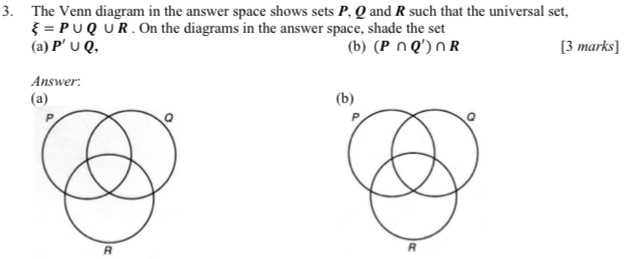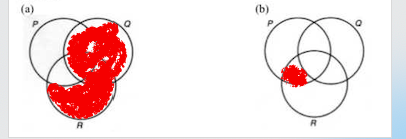### ¿Todavía tienes preguntas de matemáticas?

Pregunte a nuestros tutores expertos
Algebra
Pregunta3. The Venn diagram in the answer space shows sets $$P , Q$$ and $$R$$ such that the universal set,

$$\xi = P \cup Q \cup R$$ . On the diagrams in the answer space, shade the set

(a) $$P ^ { \prime } \cup Q$$ , (b) $$( P \cap Q ^ { \prime } ) \cap R$$